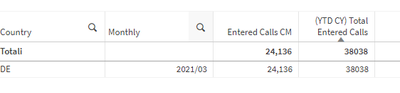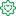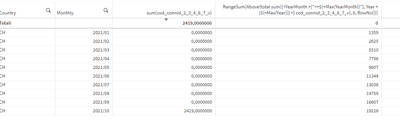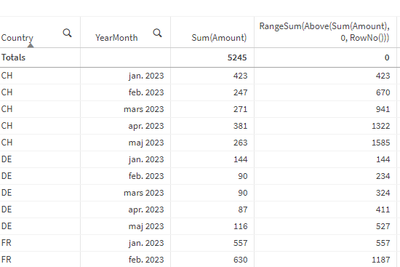Announcements
Applications are open for the 2024 Qlik Luminary Program. Apply by December 15 here.
cancel
Showing results for
Did you mean:Creator

## Sorting Problem with aggr

Hi everyone,

i have this set expression:

``aggr(RangeSum(Above(total sum({<YearMonth ={"<=\$(=Max(YearMonth))"}, Year = {\$(=Max(Year))} >} cod_connid_2_3_4_6_7_v), 0, YearMonth)),Country,YearMonth)``

which aggregates a YTD expression and give to me this result (YTD CY Total Entered Calls)Now, im trying to achieve the same results but splitted in YearWeek division, using this set expression

``aggr(RangeSum(Above(total sum({<YearMonth ={"<=\$(=Max(YearMonth))"}, Year = {\$(=Max(Year))} >} cod_connid_2_3_4_6_7_v), 0, RowNo())),Country,Yearweek)``

Anyway,even the numbers are correct, are splitted anomallyThe correct result should be thisAs you can see, sorting will not help because the number are associated to wrong dimension.

i can't figure out where the problem is, any idea?

Labels (3)

• ### Set Analysis

1 Solution

Accepted SolutionsCreator
Author

Hi!

I managed to solve in this way

``aggr(RangeSum(Above(total sum({<YearMonth ={"<=\$(=Max(YearMonth))"}, Year = {\$(=Max(Year))} >} cod_connid_2_3_4_6_7_v), 0, RowNo())),Country,(Yearweek,TEXT,ASCENDING))``

and it's working !

4 RepliesFormer Employee

The Aggr() function generates an array of values that have its own sort order - different from the sort order of the visible table. To sort the Aggr() array, you need a structured parameter. See https://help.qlik.com/en-US/qlikview/May2022/Subsystems/Client/Content/QV_QlikView/ChartFunctions/ag...

But why do you use the Aggr() at all? If you just use the RangeSum() measure, this will calculated from the visible table - which is what you want. So, try this instead:

RangeSum(Above(total sum({<YearMonth ={"<=\$(=Max(YearMonth))"}, Year = {\$(=Max(Year))} >} cod_connid_2_3_4_6_7_v), 0, RowNo(total)))Creator
Author

hi hic,

if i use you formula it always give me previous monthso that's why i use the aggr().Former Employee

Oh yes, a solution with RangeSum() does indeed work. See image below, where you clearly can see that it accumulates correctly.

If you don't get the result you want, I think you should try to find the error instead of using Aggr(). Aggr() is slower and could cause other problems.Creator
Author

Hi!

I managed to solve in this way

``aggr(RangeSum(Above(total sum({<YearMonth ={"<=\$(=Max(YearMonth))"}, Year = {\$(=Max(Year))} >} cod_connid_2_3_4_6_7_v), 0, RowNo())),Country,(Yearweek,TEXT,ASCENDING))``

and it's working !Tags
Community Browser